## Enumerating Primes

The Sieve of Eratosthenes is an efficient way to enumerate all primes up to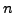. The sieve works by first writing down all numbers up to, noting that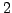is prime, and crossing off all multiples of. Next, note that the first number not crossed off is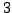, which is prime, and cross off all multiples of, etc. Repeating this process, we obtain a list of the primes up to. Formally, the algorithm is as follows:

Algorithm 1.2 (Sieve of Eratosthenes)   Given a positive integer, this algorithm computes a list of the primes up to.
1. [Initialize] Let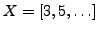be the list of all odd integers betweenand. Let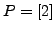be the list of primes found so far.
2. [Finished?] Let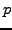be the first element of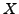. If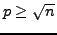, append each element ofto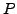and terminate. Otherwise appendto.
3. [Cross Off] Setequal to the sublist of elements inthat are not divisible by. Go to step 2.

For example, to list the primes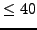using the sieve, we proceed as follows. Firstand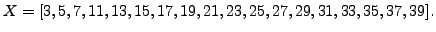We appendtoand cross off all multiples ofto obtain the new list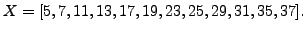Next we append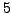to, obtaining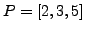, and cross off the multiples of, to obtainBecause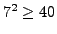, we appendtoand find that the primes less than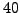are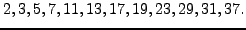Proof. [Proof of Algorithm 1.2.3] The part of the algorithm that is not clear is that when the first element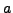ofsatisfies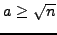, then each element ofis prime. To see this, suppose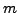is in, so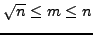and thatis divisible by no prime that is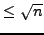. Write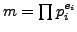with the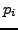distinct primes ordered so that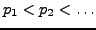. If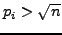for each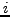and there is more than one, then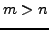, a contradiction. Thus someis less than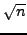, which also contradicts our assumptions on.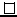SAGE Example 1.2   The eratosthenes command implements the sieve in SAGE:
sage: eratosthenes(50)
[2, 3, 5, 7, 11, 13, 17, 19, 23, 29, 31, 37, 41, 43, 47]


William 2007-06-01